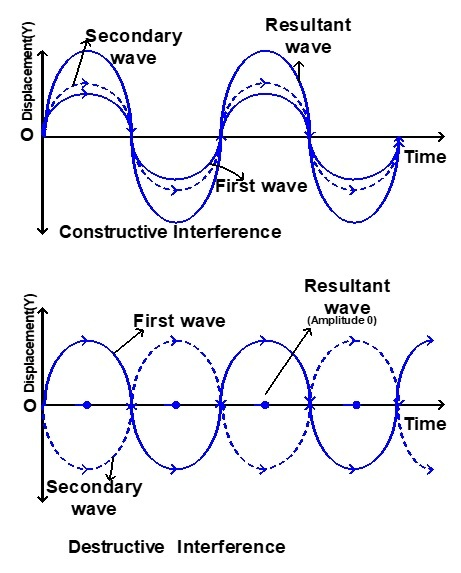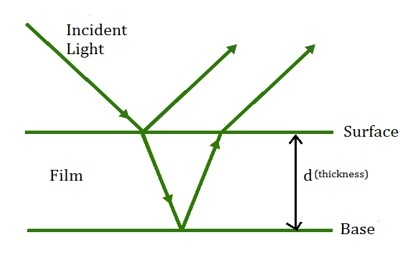# Conditions for Interference

## Introduction

Conditions for interference are one of the most important concepts of wave optics. The phenomena of light, reflection, and refraction can be explained only on the basis of wave optics. The direction in which the light propagates in the form of a wave is indicated by the light screen itself. When a stone is dropped on a motionless surface of the water, ripples spread around the area where the stone falls. This event is a perfect example of a wave spreading. As the ripple passes a particular point, the water molecules or particles at that point move up and down (or oscillate). All nodes of a wave equidistant from a focal point have a wavefront that resonates in phase.

## Interference

• When two light waves overlap each other, the phenomenon of light intensity increasing at some points and the light intensity decreasing at other points is called interference of light. Superposition refers to the addition of light waves.

• When two waves travel through a medium simultaneously the resultant displacement is equal to the vector sum of the individual displacements exerted on them by each wave.

• Depending on the phase difference between the corresponding waves, the resultant displacement can be maximum or minimum.

These concepts also apply to light. Consider light waves from two light sources $\mathrm{S_1}$ and $\mathrm{S_2}$ which meet at a point P.

A wave from source $\mathrm{S_1}$ reaches P at time t,

$$\mathrm{y_1=a_1\:sin\:ωt\:\:\:\:\:\rightarrow (1)}$$

A wave from source $\mathrm{S_2}$ reaches P at time t,

$$\mathrm{y_2=a_2 sin (\omega t+\phi)\:\:\:\:\:\rightarrow(2)}$$

These two waves have different amplitudes $\mathrm{a_1}$ and $\mathrm{a_2}$ and similar angular frequency ω and phase difference ϕ. The resultant displacement caused by these two waves

$$\mathrm{y=y_1+y_2=a_1 sin ωt+a_2 sin (\omega t+\phi)\:\:\:\:\:\rightarrow(3)}$$

Solving this equation using trigonometric identities gives the following equation,

$$\mathrm{y=A sin (\omega t+\theta)\:\:\:\:\:\rightarrow(4)}$$

Where

$$\mathrm{A=\sqrt{a_1^2+a_2^2+2a_1 a_2\:cos\:\phi}\:\:\:\:\:\rightarrow(5)}$$

$$\mathrm{θ=tan^{-1}\frac{a_2\:sin\phi}{a_1+a_2 cos\phi}}$$

The resultant amplitude is maximum at the condition $\mathrm{\phi=0,\pm 2\pi,\pm 4\pi,...}$

$$\mathrm{A_{max}=\sqrt{(a_1+a_2 )^2}}$$

The resultant amplitude is minimum at the condition $\mathrm{\phi =\pm \pi,\pm 3\pi,\pm 5\pi,...}$

$$\mathrm{A_{max}=\sqrt{(a_1-a_2 )^2}}$$

Intensity is proportional to the square of the amplitude

$$\mathrm{I \propto A^2\: \:\:\:\:\:\rightarrow(6)}$$

From equation (5)

$$\mathrm{I=I_1+I_2+2\sqrt{(I_1 I_2 )}cos \phi \:\:\:\:\:\:\rightarrow(7)}$$

## Types of Interference

### Constructive Interference

• When the crest of one wave overlaps with the crest of another wave, their amplitudes are added, creating a constructive interference and the amplitude is greater than the amplitudes of the individual waves.

• If constructive interference occurs at a point, the intensity at that point will be greater.### Destructive Interference

When the crest of one wave overlaps with the trough of another wave, destructive interference occurs. At the point where destructive interference occurs, the intensity is minimum.

## Conditions for Interference

• In equation $\mathrm{I=I_1+I_2+2\sqrt{(I_1 I_2 )}cos \phi}$, phase difference $\mathrm{\phi=0,\pm 2π,\pm 4π,...}$ is the condition for the maximum intensity of light. If the two waves overlap at this phase difference constructive interference occurs.

• In equation $\mathrm{I=I_1+I_2+2\sqrt{(I_1 I_2 )}cos\: \phi}$, phase difference $\mathrm{\phi=\pm π,\pm 3π,\pm 5π,...}$ is the condition for the minimum intensity of light.

• If $\mathrm{a_1=a_2=a}$ the equation $\mathrm{A=\sqrt{a_1^2+a_2^2+2a_1 a_2 cos \:\phi}}$ becomes

$$\mathrm{A=\sqrt{2a^2+2a^2 cos \phi}=\sqrt{2a^2 (1+cos\phi\: ϕ}}$$

$$\mathrm{A=\sqrt{2a^2 2cos^2 (\phi /2)}}$$

$$\mathrm{A=2acos(\phi /2)}$$

$$\mathrm{I\propto 4a^2 cos^2 (\phi/2)\:\:\:\:[I\propto A^2]}$$

$$\mathrm{I=4I_0cos^2 (\phi/2)\:\:\:\:[I_0\propto a^2]}$$

If $\mathrm{I_{max}=4I_0, ϕ=0,±2π,±4π .....,}$

If $\mathrm{I_{max}=0, ϕ=±π,±3π,±5π .....,}$

From this, the phase difference ϕ between the two light waves determines the intensity at the point of intersection.

## Interference in thin Films

• Consider a thin film of refractive index μ and thickness d. A parallel ray of light is fall on the thin film at an incident angle i.

• At this incident point of light, it is divided into two parts, the reflecting part and the refracting part.

• The refracted region goes into the thin film and further splits into two parts at the base of the thin film.

• Another part reflects inside the thin film. As the thin film is internally reflected multiple times, more reflective and refracted areas are formed. Light waves reflected and transmitted through thin film produce separate interference.## Interference in thin Films

### Interference by the Transmitted Light

Transmitted light waves cause interference and give the resultant intensity. The path difference will $\mathrm{\delta =2\mu d}$

The condition for constructive interference due to transmitted light is

$$\mathrm{2\mu d =n\lambda}$$

Similarly,the condition for destructive interference due to transmitted light is

$$\mathrm{2\mu d =(2n-1)\frac{\lambda}{2}}$$

### Interference by the reflected Light

It has been proved both theoretically and experimentally that light travelling through rarer medium reflected by dense medium have a phase difference of π.

The reflected light must have an additional path difference of $\mathrm{\frac{λ}{2}}$. The path difference will be $\mathrm{\delta =2\mu d}$

The condition for constructive interference by reflected light is

$$\mathrm{2μd+\frac{λ}{2}=nλ}$$

The condition for destructive interference by reflected light is

$$\mathrm{2μd+\frac{λ}{2}=(2n+1)\frac{λ}{2}}$$

## Interference in Polychromatic Light

• Interference performed in polychromatic light (White light) causes lines of different colours to appear on the screen.

• This is because different colours have different wavelengths. However, the central line is always bright and white in colour.

• This is because all colours falling at the centre O have zero path difference. Therefore, for all colours, only the constructive interference occurs at point O and the centre appears bright.

## Conclusion

The phenomena of light, reflection and refraction can be explained only on the basis of wave optics. When two light waves overlap each other, the phenomenon of light intensity increasing at some points and the light intensity decreasing at other points is called interference of light. Superposition refers to the addition of light waves. Interference performed in polychromatic light (White light) causes lines of different colours to appear on the screen. This is because different colours have different wavelengths. If constructive interference occurs at a point, the intensity at that point will be greater.

## FAQs

Q1. Explain the scattering of light

Ans. Scattering or scattering of electromagnetic radiation refers to the scattering of light waves in unexpected directions as they travel through a light medium, through defects in the medium or at the interface with another medium.

Q2. What is negative refraction?

Ans. If the permeability and penetrability of a material medium with directional and uniform properties have negative values, the light refraction in such a medium will be negative, unlike metamaterials.

Q3. What is diffraction?

Ans. The phenomenon of a wave bending along the edge of an obstacle and moving into the geometrical shadow of the obstacle is called diffraction. Diffraction is a common property of all waves including sound waves.

Q4. Why do oil films and soap bubbles on water exhibit fascinating colours?

Ans. The reason for these colours is the interference of the white light rays that are reflected multiple times between the surface and the base of the thin film. These colours depend on the angle of incidence of light, the refractive index of the film and the thickness of the film.

Q5. Define Coherent Sources

Ans. For two wave sources to be coherent sources, they must produce waves with the same phase difference or the same phase. Also, those two wave sources must produce waves of the same frequency or wavelength (monochromatic).

Updated on: 28-Apr-2023

356 Views